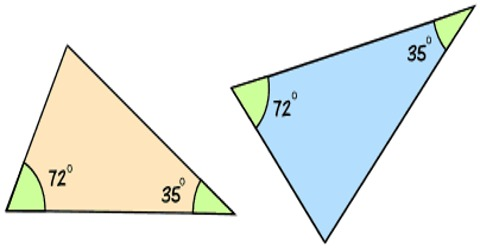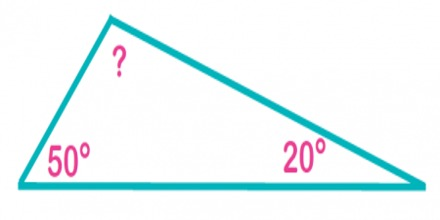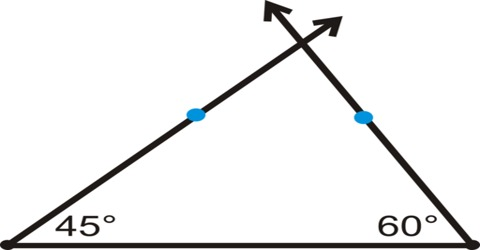Mathematic

# How to Find the Third Angle of a Triangle?Finding the Third Angle of a Triangle

Finding the third angle of a triangle when you know the measurements of the other two angles is easy.

The sums of the interior angles of a triangle are equal to 1800. To find the third angle of a triangle when the other two angles are known subtract the number of degrees in the other two angles from 1800.All you’ve got to do is subtract the other angle measurements from 180° to get the measurement of the third angle. However, there are a few other ways to find the measurement of the third angle of a triangle, depending on the problem you’re working with.

Example: How many degrees are in the third angle of a triangle whose other two angles are 450 and 600?Solution:

The three angles of any triangle always add up to be 180.

So if x, y, and z are the three angles, then x + y + z = 180.

If z is the third angle, then z = 180 – x – y.

Another way to look at this is that you start with 180 and you subtract away the two angles you know, x and y, which leaves you an amount for the third angle.

Answer: 1800 – 450 – 600 = 750